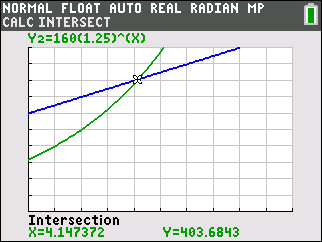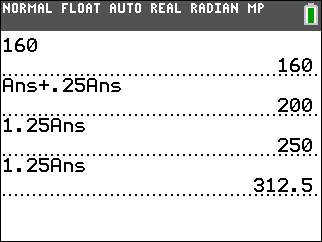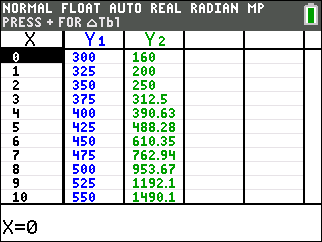••• ##### Device
• TI-84 Plus
• TI-84 Plus Silver Edition
•TI-84 Plus C Silver Edition
•TI-84 Plus CE
• ##### Report an Issue

Middle Grades Math: Guppies and Frogs
by Texas Instruments#### Overview

In this activity, students will create equations with two or more variables to represent relationships between quantities. They will also graph equations on coordinate axes with labels and scales.

#### Key Steps

•Students will write functions that describe a linear and exponential relationship between two quantities.

•Students will interpret and compare the average rate of change of a function with the average percent change of a function.

•Students will analyze the equations and graphs of linear and exponential models to determine properties of the models.